Search IntMath
Close

450+ Math Lessons written by Math Professors and Teachers

5 Million+ Students Helped Each Year

1200+ Articles Written by Math Educators and Enthusiasts

Simplifying and Teaching Math for Over 23 Years

# IntMath Newsletter: Eigenvectors, 3D solar system, graphics

By Murray Bourne, 30 Jul 2019

30 Jul 2019

1. New on IntMath: Eigenvalues and eigenvectors
2. Resources: Desmos and 3D simulator
3. Math in the news: News and science
4. Math movies: Graphics and addresses
5. Math puzzle: Tangent circles
6. Final thought: Culture

## 1. New on IntMath: Eigenvalues and eigenvectors

Eigenvectors are very important in many science and engineering fields, including compuer science. (Google's search algorithm is based on eigenvectors.) It was one of the many topics I learned about as a math student where I could find the answers, but I really had no idea what I'd found, what the concept really meant, or what they were good for.

I wrote some new pages incorporating interactive applets which I hope gives the reader a better idea of what this interesting topic is all about. (It's usually taught at university level, but the mathematics involved is not that challenging - mostly multiplying and adding.)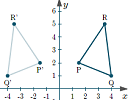On this page we see how multiplying by a matrix can achieve the kind of transformations graphics artists and robot designers use a lot, including reflection, rotation, skew (shear), scaling and displacement.

The applet on this next page allows you to explore the physical meaning and geometric interpretation of eigenvalues and eigenvectors.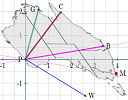I've used stretching of a map of my home country to show how transformations achieved by matrix multiplication can be replaced with much simpler scalar multiplication (which is a key idea of this topic).

Like most matrix operations, it's very easy to make simple mistakes when calculating eigenvalues and eigenvectors.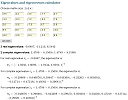This calculator allows you to check your work, and/or to explore what happens with eigenvectors for everything from 2x2 and 3x3 up to 9x9-size matrices.

You may also be interested in:

How to find eigenvalues and eigenvectors? (outlining the algebraic steps for finding them - this was the only part I learned at university).

Applications of eigenvalues and eigenvectors (which includes a highly simplified description of how Google search works).

## 2. Resources

### (a) Using Desmos to create learning resources

Here's a recent tweet by teacher Liz Caffrey: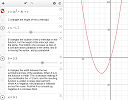Check out how one of my students uses the graphing calculator to take notes! She sets up and saves a new graph each day. Here are her notes from our notice/wonder on standard form of quadratics! I never would have thought of doing this. Math Classwork 4/23/19 - Trinomial Explorations

I know from my own experience how much richer my understanding of topics is after I've created similar interactive explorations. Doing it with Desmos is really quite easy, and I encourage you (whether student or teacher) to give it a go. You don't need a lot in the way of programming skills.

### (b) Harmony of the spheres

This is a 3D simulation of the solar system, built on an "open source gravity simulator".

Earth and Moon system

It also has some "What-if" scenarios. This example shows what would happen if Earth actually orbitted Saturn: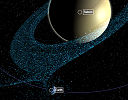You can drag left-righ, up-down to explore and use the mouse wheel to zoom. Press the "Play" button at the top to animate the scene.

You can also change the physics parameters, and add masses. It's very nicely done. (Probably best on a laptop.)

## 3. Math in the news: News and science

Last week marked the 50th anniversary of the moon landings. This was an extraordinary human achievement, but the misguided belief that it was all a hoax continues to grow, fuelled by ignorant people (and bots) through social media. Where and how we find our news, and how we critically examine the sources and conclusions, becomes increasingly important as the growth of fake news has the potential to rip apart our societies.

### (a) How do we find out what's happening?

In the US, more people get their news from social media than traditional news sources, while in other countries it's the reverse. See a short summary: Where do people find news on their smartphones?

From the original Digital News Report, by Reuters and University of Oxford: Executive Summary and Key Findings of the 2019 Report

### (b) What do we know about science?

Those of us involved in science education would like to think most students graduate with a reasonable general knowledge of the various branches of science, and will have a good grounding in how science actually works. But it appears that is not always the case.

The (US) National Science Board's Science and Engineering Indicators 2018 makes for some sobering reading.

Science and Technology: Public Attitudes and Understanding

Here's a sample of the misunderstandings gleaned from the results of various quizzes.

• Antibiotics kill viruses (which is false): 50% or more got it wrong in most countries
• Human beings developed from earlier species of animals (true): 30 to 50% got it wrong
• Astrology is scientific (false): around 40% (US) thought it was true
• Understanding experimental design: over 50% got it wrong in most countries

No wonder we have the growth of drug-resistant superbugs, rampant species destruction, and climate change deniers.

## 4. Math Movies

### (a) The simple genius of a good graphic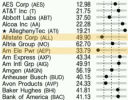Data visualization is an increasingly important item in everyone's skill set these days. Information designer Tommy McCall outlines the evolution of charts and diagrams with plenty of interesting examples.

### (b) A precise, three-word address for every place on Earth

When I first arrived in Japan where I lived for 4 years, I was amazed at how different their address system was compared to what Iwas used to. Rather than sequential numbers (usually odd on one side and even on the other) along roads, the Japanese system is built around regions and sub-regions of a suburb.

So I found the pretext of this next talk quite interesting.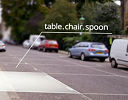Many houses do not have any kind of address system, and to find anyone, you need to rely on local knowledge. Chris Sheldrick has an interesting solution - use three-word addresses!

I like the basic concept, but surely numbers are more universal? Wouldn't addresses based on latitude and longitude make more sense, especially as that's how our phones know where we are already?

## 5. Math puzzles

A correct answer with sufficient reasons was submitted by Georgios. Special mention to Nicola who worked on a generalized solution involving circles and ellipses, which she explored using Desmos.

### New math puzzle: Tangent circles

Circles that are "mutually tangent" touch at one point only. We have 3 such circles, with centers P, Q, R and radii p, q, r respectively. We are given the lengths PQ = 15, QR = 21 and PR = 14. Find p, q and r.

You can leave your response here.

## 6. Final thought - culture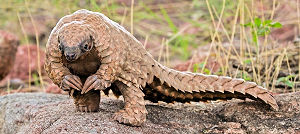Pangolin (image credit)

Living in different countries gives you fresh insights about culture. A lot of the cultural practices that seemed vital to our existence have faded as time goes on, and others we question due to their environmental impacts. While they may not have had much effect hundreds of years ago when there were less people in the world, perhaps some aspects of the following don't make a lot of sense any more?

• Living in large houses a long way from work
• Eating whale meat
• Burning paper in the Hungry Ghost Festival
• Consuming rhino horn, pangolin scales and shark fin
• Wearing baby seal furs

Maintaining the traditions while minimizing their impacts is surely the best way to move forward.

Until next time, enjoy whatever you learn.

1. Bob says:

Mobile phones are not reliable for location of one's house, at least those with a map from a prominent map publisher, to judge by the mobile-phone users who end up at the wrong address when coming to our place. Latitude and Longitude as an address; would it not perhaps depend on the geoid of the day? As well as the functionality of the satellites, access to a battery charger from time to time and the trust that one's mobile phone has not been stolen or dropped or drowned or forgotten. No, as in the good old days before Microsoft, Apple, the mobile phone, ebay, 24/7 shopping, ATMs and credit cards, life was simpler, less expensive, less fraught and indeed a lot easier, particularly when one was guaranteed the arrival of letters the day after their posting in either the first or second delivery of the day, nails were sold by the pound in a brown paper bag at the ironmonger's and milk was delivered in glass bottles with the cream on top. I'll stick with numbers and the street's name; they work very well.Like clay tablets with Sumerian script for data storage.

2. r. m. luther says:

Misprint PQ=15 and PQ=14

3. Murray says:

@r.m.luther: Thank you so much! I have amended the post - rather too late since the mail has already gone out. Hopefully others will also realise what it was meant to be.

4. Kathy says:

You have stated two different values for PQ.

5. Murray says:

@Kathy: Sorry about the typo. It should have been PR = 14 at the end (not PQ).

6. Arundhati Mukherjee says:

4 , 11 and 10

7. Russell Wild says:

I think a small typo crept into the latest problem.

Circles that are "mutually tangent" touch at one point only. We have 3 such circles, with centers P, Q, R and radii p, q, r respectively. We are given the lengths PQ = 15, QR = 21 and PQ = 14. Find p, q and r.

Surely PR=14, not PQ.

Or have I missed something?
Regards

8. Murray says:

@Russell: Indeed, I have a typo and you surmise correctly. I've amended the post.

9. Russell Wild says:

From information given
p+q=15 (1)
p+r=14 (2)
r+q=21 (3)

Subtract (2) from (1)
then q-r=1 (4)
2q=22
ie q=11
Substitute q=11 in (1)
ie p=4
Substitute q=11 in (3)
ie r=10

10. Nicola says:

This looks like a simple linear algebra problem (unless I am missing something!).
PQ=15=p+q
PR=14=p+r
QR=21=q+r

The distance between the centres is the sum of the radii because the circles are tangent.

We can solve this with substitutions or with matrix Ax=b.
1p+1q+0r=15
1p+0q+1r=14
0p+1q+1r=21

A=[1 1 0
1 0 1
0 1 1]
b=[15 14 21]^T

We take the inverse of A, A^-1, and multiply it by the b vector.

The result is a vector with p=4, q=11, r=10.

11. Tomas Garza says:

Let PQ = 15, QR = 21 and PR = 14. Let p, q and r be the radii of the circles centered at P, Q and R, respectively. The condition for any three circles to be tangent to each other is that the distance between their centers be equal to the sum of ther radii. Then we have the three equations

p + q = 15; q + r = 21; p + r = 14

the solution of which gives

p = 4, q = 11 and r = 10

12. Thomas A Buckley says:

p=4, q=11, r=10 because:
At points of Tangent radii of both circles are
at 90 deg to tangent, so are in line through centres.

This means PQ =p+q =15, QR= q+r =21, and PR=p+r =14
( Assuming repeat of PQ, in news letter,a typo of PR !)

Then p=15-q. Substitute for p in p+r=14
15-q+r =14. => q= r+1

From q+r =21 substitute r+1 for q
Then r+1+r =21. => r= 10 .... Part Ans
As q=r+1, q=10+1 =11 .... Part Ans

From p+q =15, and q=11
So p = 15-11 =4 .... Part Ans

13. karam says:

q = 15 - p ......(1)

r = 14 - p ......(2)

q + r = 15 - p + 14 - p

21 = 29 - 2p

p = 4
from (1) q= 11
from (2) r = 10

### Comment Preview

HTML: You can use simple tags like <b>, <a href="...">, etc.

To enter math, you can can either:

1. Use simple calculator-like input in the following format (surround your math in backticks, or qq on tablet or phone):
a^2 = sqrt(b^2 + c^2)
(See more on ASCIIMath syntax); or
2. Use simple LaTeX in the following format. Surround your math with $$ and $$.
$$\int g dx = \sqrt{\frac{a}{b}}$$
(This is standard simple LaTeX.)

NOTE: You can mix both types of math entry in your comment.